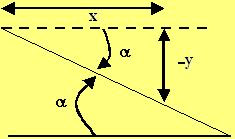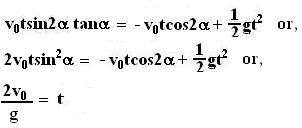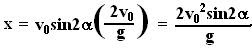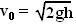## Thursday, November 13, 2014

### Irodov Problem 1.31The entire event is depicted in the figure. The ball first falls from a height h on to the inclined plane and then reflects off of the plane. The ball will hit the plane at an angleand thus will at an angle that forms an equal anglewith the normal to the plane. Thus, the ball will reflect off of the inclined plane at an angle ofwith the horizontal as shown in the figure.

Let the point of contact of the ball with the inclined plane for the first time be the origin in our Cartesian coordinate system and let t=0. Then the position of the ball after reflection at any time is given by,Here V0 is the velocity at first impact on the inclined plane.Now this ball must meet the inclined plane. Any point on the inclined plane satisfies the relationThis can be seen from the figure drawn beside.

Thus where ever the ball meets the plane, the inclined plane relation must also hold true. In other words,Now knowing the time we can calculate the coordinate of where the ball hits the inclined plane as,Now the distance traveled by the ball along the inclined plane is given as,Since the ball fell a height h before hitting the inclined plane, it must have attained a velocity of. This relation can be obtained by either equating the potential energy lost by the balls (mgh) during the fall and equating to its kinetic energy upon impact (½ mv02) . Now substituting this value of initial velocity in to our found value of l we have,## Sunday, November 9, 2014

### TutorState.com 718-223-0228

Chapter 21, Problem 4.
A single loop of wire with an area of x1 m2 is in a uniform magnetic field that has an initial value of x2 T, is perpendicular to the plane of the loop, and is decreased with the constant rate of x3 T/s.
(a) What emf is induced in this loop?
(b) If the loop has a resistance of x4 Ω, find the current induced in the loop.

Chapter 21, problem 7.
A closely wound rectangular coil of N turns has dimensions of x1 cm by x2 cm. The plane of the coil is rotated from a position where it makes an angle of x3ᵒ with a magnetic field of x4 T to a position perpendicular to the field. The rotation takes x5 s.
What is average emf induced on the coil?http://tutorstate.blogspot.com/